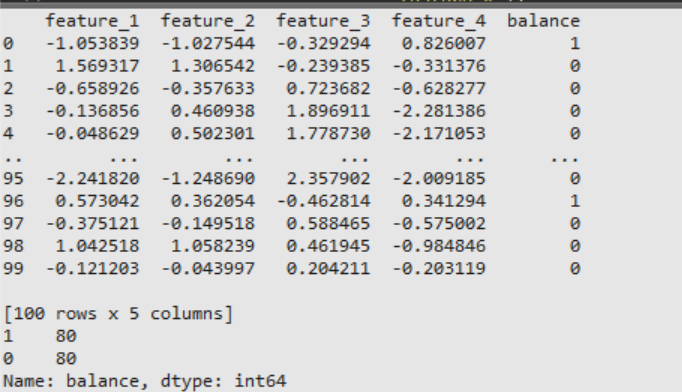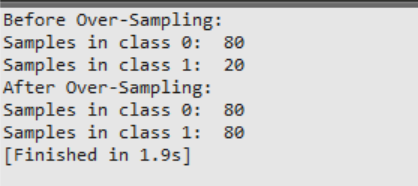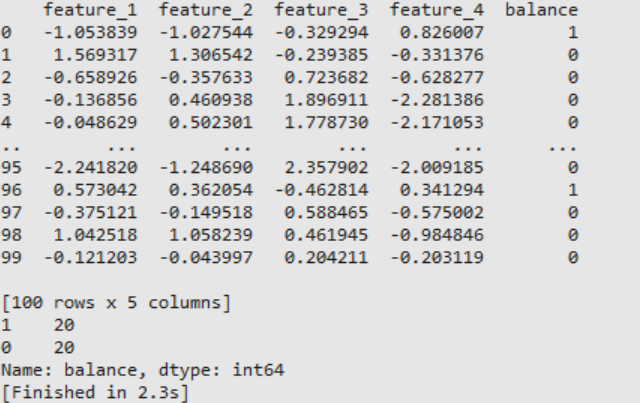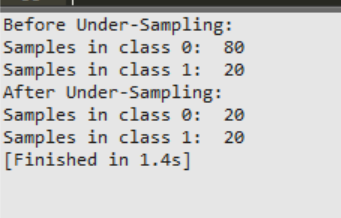# How to Handle Imbalanced Classes in Machine Learning

In machine learning, “imbalanced classes” is a familiar problem particularly occurring in classification when we have datasets with an unequal ratio of data points in each class. Training of model becomes much trickier as typical accuracy is no longer a reliable metric for measuring the performance of the model. Now if the number of data points in minority class is much less, then it may end up being completely ignored during training.

## Over/Up-Sample Minority Class

In Up-sampling, samples from minority classes are randomly duplicated so as to achieve equivalence with the majority class. There are many methods used for achieving this.

### 1. Using scikit-learn:

This can be done by importing resample module from scikit-learn.

Syntax: sklearn.utils.resample(*arrays, replace=True, n_samples=None, random_state=None, stratify=None)

Parameters:

• *arrays: Dataframe/lists/arrays
• replace: Implements resampling with or without replacement. Boolean type of value. Default value is True.
• n_samples: Number of samples to be generated. Default value is None. If value is None then first dimension of array is taken automatically. This value will not be longer than length of arrays if replace is given as False.
• random_state: Used for shuffling the data. If positive non zero number is given then it shuffles otherwise not. Default value is None.
• stratify: Data is split in stratified fashion if set to True. Default value is None.

Return Value: Sequence of resampled data.

Example :

## Python3

 `# Importing scikit-learn, pandas library ` `from` `sklearn.utils ``import` `resample ` `from` `sklearn.datasets ``import` `make_classification ` `import` `pandas as pd ` ` `  `# Making DataFrame having 100 ` `# dummy samples with 4 features  ` `# Divided in 2 classes in a ratio of 80:20  ` `X, y ``=` `make_classification(n_classes``=``2``,  ` `                           ``weights``=``[``0.8``, ``0.2``], ` `                           ``n_features``=``4``,  ` `                           ``n_samples``=``100``,  ` `                           ``random_state``=``42``) ` ` `  `df ``=` `pd.DataFrame(X, columns``=``[``'feature_1'``, ` `                              ``'feature_2'``, ` `                              ``'feature_3'``, ` `                              ``'feature_4'``]) ` `df[``'balance'``] ``=` `y ` `print``(df) ` ` `  `# Let df represent the dataset ` `# Dividing majority and minority classes ` `df_major ``=` `df[df.balance ``=``=` `0``] ` `df_minor ``=` `df[df.balance ``=``=` `1``] ` ` `  `# Upsampling minority class ` `df_minor_sample ``=` `resample(df_minor, ` `                            `  `                           ``# Upsample with replacement ` `                           ``replace``=``True``,     ` `                            `  `                           ``# Number to match majority class ` `                           ``n_samples``=``80``,    ` `                           ``random_state``=``42``) ` ` `  `# Combine majority and upsampled minority class ` `df_sample ``=` `pd.concat([df_major, df_minor_sample]) ` ` `  `# Display count of data points in both class ` `print``(df_sample.balance.value_counts())`

Output:Explanation :

• Firstly, we’ll divide the data points from each class into separate DataFrames.
• After this, the minority class is resampled with replacement by setting the number of data points equivalent to that of the majority class.
• In the end, we’ll concatenate the original majority class DataFrame and up-sampled minority class DataFrame.

### 2. Using RandomOverSampler:

This can be done with the help of the RandomOverSampler method present in imblearn. This function randomly generates new data points belonging to the minority class with replacement (by default).

Syntax: RandomOverSampler(sampling_strategy=’auto’, random_state=None, shrinkage=None)

Parameters:

• sampling_strategy: Sampling Information for dataset.Some Values are- ‘minority’: only minority class ‘not minority’: all classes except minority class, ‘not majority’: all classes except majority class, ‘all’: all classes,  ‘auto’: similar to ‘not majority’, Default value is ‘auto’
• random_state: Used for shuffling the data. If a positive non-zero number is given then it shuffles otherwise not. Default value is None.
• shrinkage: Parameter controlling the shrinkage. Values are: float: Shrinkage factor applied on all classes. dict: Every class will have a specific shrinkage factor. None: Shrinkage= 0. Default value is None.

Example:

## Python3

 `# Importing imblearn,scikit-learn library ` `from` `imblearn.over_sampling ``import` `RandomOverSampler ` `from` `sklearn.datasets ``import` `make_classification ` ` `  `# Making Dataset having 100 ` `# dummy samples with 4 features  ` `# Divided in 2 classes in a ratio of 80:20  ` `X, y ``=` `make_classification(n_classes``=``2``,  ` `                           ``weights``=``[``0.8``, ``0.2``], ` `                           ``n_features``=``4``,  ` `                           ``n_samples``=``100``,  ` `                           ``random_state``=``42``) ` ` `  `# Printing number of samples in ` `# each class before Over-Sampling ` `t ``=` `[(d) ``for` `d ``in` `y ``if` `d``=``=``0``] ` `s ``=` `[(d) ``for` `d ``in` `y ``if` `d``=``=``1``] ` `print``(``'Before Over-Sampling: '``) ` `print``(``'Samples in class 0: '``,``len``(t)) ` `print``(``'Samples in class 1: '``,``len``(s)) ` ` `  `# Over Sampling Minority class ` `OverS ``=` `RandomOverSampler(random_state``=``42``) ` ` `  `# Fit predictor (x variable) ` `# and target (y variable) using fit_resample() ` `X_Over, Y_Over ``=` `OverS.fit_resample(X, y) ` ` `  `# Printing number of samples in ` `# each class after Over-Sampling ` `t ``=` `[(d) ``for` `d ``in` `Y_Over ``if` `d``=``=``0``] ` `s ``=` `[(d) ``for` `d ``in` `Y_Over ``if` `d``=``=``1``] ` `print``(``'After Over-Sampling: '``) ` `print``(``'Samples in class 0: '``,``len``(t)) ` `print``(``'Samples in class 1: '``,``len``(s)) `

Output:### 3. Synthetic Minority Oversampling Technique (SMOTE):

SMOTE is used to generate artificial/synthetic samples for the minority class. This technique works by randomly choosing a sample from a minority class and determining K-Nearest Neighbors for this sample, then the artificial sample is added between the picked sample and its neighbors. This function is present in imblearn module.

Syntax: SMOTE(sampling_strategy=’auto’, random_state=None, k_neighbors=5, n_jobs=None)

Parameters:

• sampling_strategy: Sampling Information for dataset
• random_state: Used for shuffling the data. If positive non zero number is given then it shuffles otherwise not. Default value is None.
• k_neighbors: Number count of nearest neighbours used to generate artificial/synthetic samples. Default value is 5
• n_jobs: Number of CPU cores to be used. Default value is None. None here means 1 not 0.

Example:

## Python3

 `# Importing imblearn, scikit-learn library ` `from` `imblearn.over_sampling ``import` `SMOTE ` `from` `sklearn.datasets ``import` `make_classification ` ` `  `# Making Dataset having ` `# 100 dummy samples with 4 features  ` `# Divided in 2 classes in a ratio of 80:20  ` `X, y ``=` `make_classification(n_classes``=``2``,  ` `                           ``weights``=``[``0.8``, ``0.2``], ` `                           ``n_features``=``4``,  ` `                           ``n_samples``=``100``,  ` `                           ``random_state``=``42``) ` ` `  `# Printing number of samples in ` `# each class before Over-Sampling ` `t ``=` `[(d) ``for` `d ``in` `y ``if` `d``=``=``0``] ` `s ``=` `[(d) ``for` `d ``in` `y ``if` `d``=``=``1``] ` `print``(``'Before Over-Sampling: '``) ` `print``(``'Samples in class 0: '``,``len``(t)) ` `print``(``'Samples in class 1: '``,``len``(s)) ` ` `  ` `  `# Making an instance of SMOTE class  ` `# For oversampling of minority class ` `smote ``=` `SMOTE() ` ` `  `# Fit predictor (x variable) ` `# and target (y variable) using fit_resample() ` `X_OverSmote, Y_OverSmote ``=` `smote.fit_resample(X, y) ` ` `  `# Printing number of samples ` `# in each class after Over-Sampling ` `t ``=` `[(d) ``for` `d ``in` `Y_OverSmote ``if` `d``=``=``0``] ` `s ``=` `[(d) ``for` `d ``in` `Y_OverSmote ``if` `d``=``=``1``] ` `print``(``'After Over-Sampling: '``) ` `print``(``'Samples in class 0: '``,``len``(t)) ` `print``(``'Samples in class 1: '``,``len``(s)) `

Output:Explanation:

• Minority class is given as input vector.
• Determine its K-Nearest Neighbours
• Pick one of these neighbors and place an artificial sample point anywhere between the neighbor and sample point under consideration.
• Repeat till the dataset gets balanced.

• No information loss
• Better than under sampling

1. Increased chances of over-fitting as duplicates of minority class is made

## Down/Under-sample Majority Class

Down/Under Sampling is the process of randomly selecting samples of majority class and removing them in order to prevent them from dominating over the minority class in the dataset.

### 1. Using scikit-learn :

It is similar to up-sampling and can be done by importing resample module from scikit-learn.

Example :

## Python3

 `# Importing scikit-learn, pandas library ` `from` `sklearn.utils ``import` `resample ` `from` `sklearn.datasets ``import` `make_classification ` `import` `pandas as pd ` ` `  `# Making DataFrame having ` `# 100 dummy samples with 4 features  ` `# Divided in 2 classes in a ratio of 80:20  ` `X, y ``=` `make_classification(n_classes``=``2``, ` `                           ``weights``=``[``0.8``, ``0.2``], ` `                           ``n_features``=``4``,  ` `                           ``n_samples``=``100``,  ` `                           ``random_state``=``42``) ` ` `  `df ``=` `pd.DataFrame(X, columns``=``[``'feature_1'``, ` `                              ``'feature_2'``, ` `                              ``'feature_3'``, ` `                              ``'feature_4'``]) ` `df[``'balance'``] ``=` `y ` `print``(df) ` ` `  `# Let df represent the dataset ` `# Dividing majority and minority classes ` `df_major ``=` `df[df.balance``=``=``0``] ` `df_minor ``=` `df[df.balance``=``=``1``] ` `  `  `# Down sampling majority class ` `df_major_sample ``=` `resample(df_major, ` `               ``replace``=``False``,  ``# Down sample without replacement ` `               ``n_samples``=``20``,   ``# Number to match minority class ` `               ``random_state``=``42``) ` ` `  `# Combine down sampled majority class and minority class ` `df_sample ``=` `pd.concat([df_major_sample, df_minor]) ` `  `  `# Display count of data points in both class ` `print``(df_sample.balance.value_counts()) `

Output:Explanation :

• Firstly, we’ll divide the data points from each class into separate DataFrames.
• After this, the majority class is resampled without replacement by setting the number of data points equivalent to that of the minority class.
• In the end we’ll concatenate the original minority class DataFrame and down-sampled majority class DataFrame.

### 2: Using RandomUnderSampler

This can be done with the help of RandomUnderSampler method present in imblearn. This function randomly selects a subset of data for the class.

Syntax: RandomUnderSampler(sampling_strategy=’auto’, random_state=None, replacement=False)

Parameters:

• sampling_strategy: Sampling Information for dataset.
• random_state: Used for shuffling the data. If positive non zero number is given then it shuffles otherwise not. Default value is None.
• replacement: Implements resampling with or without replacement. Boolean type of value. Default value is False.

Example:

## Python3

 `# Importing imblearn library ` `from` `imblearn.under_sampling ``import` `RandomUnderSampler ` `from` `sklearn.datasets ``import` `make_classification ` ` `  `# Making Dataset having ` `# 100 dummy samples with 4 features  ` `# Divided in 2 classes in a ratio of 80:20  ` `X, y ``=` `make_classification(n_classes``=``2``,  ` `                           ``weights``=``[``0.8``, ``0.2``], ` `                           ``n_features``=``4``,  ` `                           ``n_samples``=``100``,  ` `                           ``random_state``=``42``) ` ` `  `# Printing number of samples ` `# in each class before Under-Sampling ` `t ``=` `[(d) ``for` `d ``in` `y ``if` `d``=``=``0``] ` `s ``=` `[(d) ``for` `d ``in` `y ``if` `d``=``=``1``] ` `print``(``'Before Under-Sampling: '``) ` `print``(``'Samples in class 0: '``,``len``(t)) ` `print``(``'Samples in class 1: '``,``len``(s)) ` ` `  `# Down-Sampling majority class ` `UnderS ``=` `RandomUnderSampler(random_state``=``42``, ` `                            ``replacement``=``True``) ` ` `  `# Fit predictor (x variable) ` `# and target (y variable) using fit_resample() ` `X_Under, Y_Under ``=` `UnderS.fit_resample(X, y) ` ` `  `# Printing number of samples in ` `# each class after Under-Sampling ` `t ``=` `[(d) ``for` `d ``in` `Y_Under ``if` `d``=``=``0``] ` `s ``=` `[(d) ``for` `d ``in` `Y_Under ``if` `d``=``=``1``] ` `print``(``'After Under-Sampling: '``) ` `print``(``'Samples in class 0: '``,``len``(t)) ` `print``(``'Samples in class 1: '``,``len``(s)) `

Output:• Better run time
• Improves storage problem as training examples are reduced